## A stiff wire 50.0 cm long is bent at a right angle in the middle. One section lies along the z axis and the other is along the line y=2x in

Question

A stiff wire 50.0 cm long is bent at a right angle in the middle. One section lies along the z axis and the other is along the line y=2x in the xy plane. A current of 20.0 A flows in the wire — down the z axis and out the line in the xy plane. The wire passes through a uniform magnetic field B = (.318 i)T. Determine the magnitude and the direction of the total force on the wire.

in progress 0
2 months 2021-07-30T02:38:02+00:00 2 Answers 0 views 0

F=2.38i – 4.57j

magnitude of F=5.15N

Explanation:

The force on the wire is given by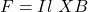in this problem we have to compute the force for both sections if the wire. Hence we have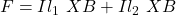The direction of l1 is k. The direction of l2 is obtained by using the slope of the line y=2x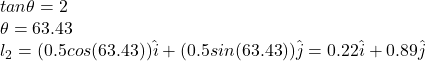By applying the cross product we have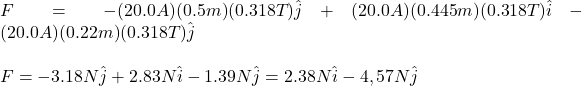and its magnitude is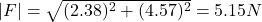HOPE THIS HELPS!!

Magnitude of the force is 2.135N and the direction is 41.8° below negative y-axis

Explanation:

The stiff wire 50.0cm long bent at a right angle in the middle

One section lies along the z axis and the other is along the line y=2x in the xy plane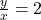tan θ = 2

Therefore,

slope m = tan θ = y / x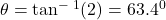Then length of each section is 25.0cm

so, length vector of the wire is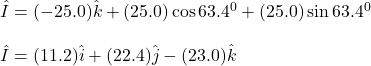And magnetic field is B = (0.318T)i

Therefore,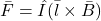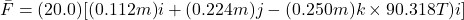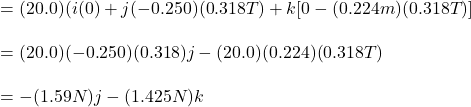Magnitude of the force is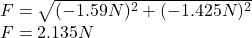Direction is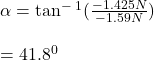Magnitude of the force is 2.135N and the direction is 41.8° below negative y-axis